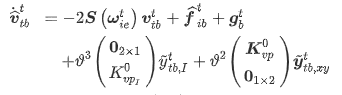# Skew symmetric 1 dimension

• B
Summary:
if I have a equation like (just a random eq.) p_dot = S(omega)*p. where p = [x, y, z] is the original states, omega = [p, q, r] and S - skew symmetric. How does the equation appear if i only want a system to have the state z?
Hi,

if I have a equation like (just a random eq.) p_dot = S(omega)*p. where p = [x, y, z] is the original states, omega = [p, q, r] and S - skew symmetric.
How does the equation appear if i only want a system to have the state z? do I get z_dot = -q*x + p*y. Or is the symmetric not valid so I simply get z_dot = z? or something else?
and the same for rotation matrix? : p_dot = R(omega)*p

Thanks for any replies!

berkeman
Mentor
(just a random eq.)
You are asking us to decode a random equation that you made up?

And it would help if you would please learn to post math equations using LaTeX. That would make your postings a lot more clear (well, maybe not if you keep posting random equations...).

The PF LaTeX tutorial is available in the Help pages, under INFO at the top of the page.

Hi berkeman. sorry, here is the complete equation with v, omega, f and g beeing 3x1 vectors:Im just curious about the first term with the skew symmetric, how it will turn out when I only have it in 1 dimension, like described in original post.

fresh_42
Mentor
What should skew symmetry mean in one dimension? S=0? I suspect from your question that we speak about quantum physics, and the three dimensional skew symmetric matrices form a semisimple Lie algebra. It's no longer semisimple in the one dimensional case which is crucial, skew symmetric or not, hence irrelevant in the context you hinted at.